### Python按位运算

• 发布时间：2016年11月24日 17:20
• 作者：杨仕航
• 分类标签： Python
• 阅读(10182)
• 评论(0)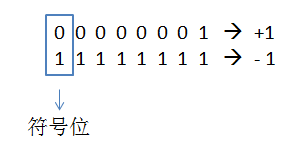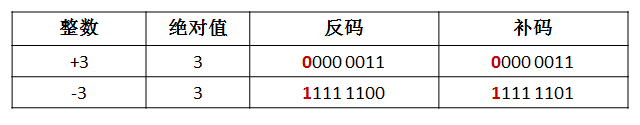1、正数的二进制值，原码、补码、反码都是一样的；

2、负数的二进制值是其补码，即反码加1。

1）按位与（&）

2）按位或（|）

3）按位异或（^）

4）按位翻转（~）

5）左移运算（<<）

6）右移运算（>>）

#### 1、按位与（&）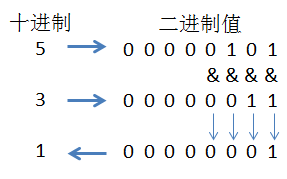5&3结果为1。

`n%3 == 1  #True为偶数，False为奇数`

`n&1 == 1  #按位于运算奇数返回1，偶数返回0`

#### 2、按位或（|）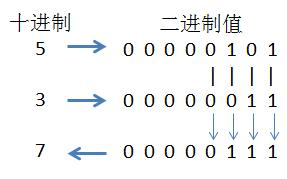5|3结果为7。

`map(lambda x:x|1, range(6))`

#### 3、按位异或（^）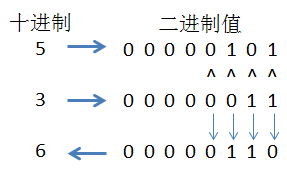5^3结果为6。

```def get_one(nums):
nums_dict = {}
for num in nums:
#遍历数字列表，并累计统计
nums_dict[num] = nums_dict.get(num, 0) + 1
for key, value in nums_dict.iteritems():
if value==1:
#返回值为1的键值
return key```

```def get_one(nums):
return reduce(lambda x,y: x^y, nums)```

#### 4、按位翻转（~）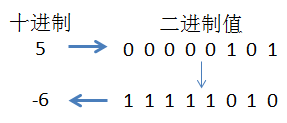#### 5、左移运算（<<）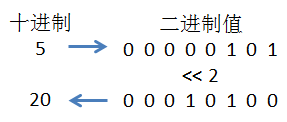```  5 * 7
= 101B * 111B
= 101B * (100B + 10B + 1B)
= 101B * 100B + 101B * 10B + 101B * 1B
= 5<<2 + 5<<1 + 5<<0
= 20 + 10 + 5
= 35```

#### 6、右移运算（>>）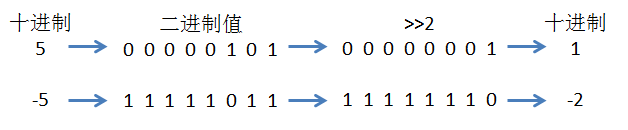ps：本道题虽然可以用math模版的log求解，但效率不是更高。可以试试按位与运算。